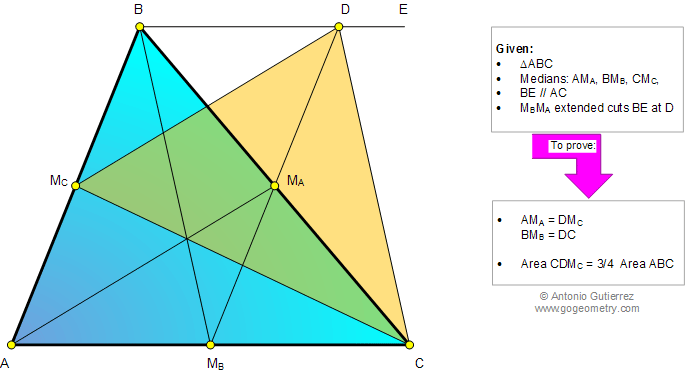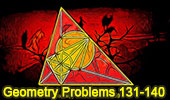###### Problem 860: Triangle, Three Medians, Parallel, Parallelogram, Area, Congruence. Level: High School, SAT Prep, College geometry
 The figure shows a triangle ABC. with the medians AMA, BMB, and CMC. BE is parallel to AC and MBMA extended cuts BE at D. Prove that (1) AMA = DMC, BMB = DC; (2) Area of the triangle CDMC is 3/4 the area of the triangle ABC.More Geometry Problems:Geometry Problem 859 Isosceles Right Triangle, Angles,18, 45 Degrees, Congruence, Perpendicular bisector. Geometry Problem 858 Isosceles Right Triangle, Cevian, Ratio 2:1, 45 Degrees, Congruence, Angles, Auxiliary Lines. Geometry Problem 857 Triangle, Median, Midpoint, Angles, 30, 45 Degrees, Congruence, Auxiliary Lines.
 Home | Search | Geometry | Problems | All Problems | Open Problems | Visual Index | 10 Problems | 851-860 | Triangles | Congruence | Triangle area | Parallel | Parallelogram | Median | Email | Solution / comment | By Antonio Gutierrez